# Le catalogue des pièces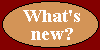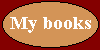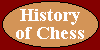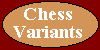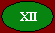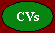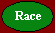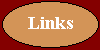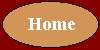Move Shako Metamachy Zanzibar Maasai Chess Teramachy Gigachess Terachess King1 step in all directions. May castle with the Rook. Cannot be put in check. X X X X X X X X QueenAll direction slider. (Rook + Bishop) X X X X X X X X Pawn1 (or 2 in some conditions) steps straight forward (no capture); captures 1 step diagonally forward. X X X X X X X X Rookslides orthogonally. X X X X X X X X Bishopslides diagonally. X X X X X X X X Knight(1,2) leaper X X X X X X X X Cannonmoves as Rook, needs interposing piece to capture X X X X X X X X Elephant1 or 2 steps diagonally. May leap. X X X X X X X X Camel(1,3) leaper X X X X X X X Eagle1 step diagonally then slide on columns or lines X X X X X X X Ship1 step diagonally then slide on columns X* X* X* Prince1 step in all directions. Move (no capture) 2 steps forward on some conditions X* X X X X X X LionMachine + Elephant + Knight X X X X X X Machine1 or 2 steps orthogonally. May leap. X X X X X Giraffe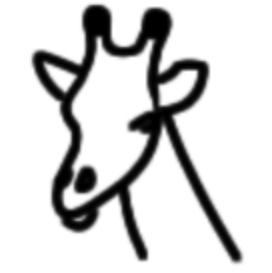(2,3) leaper X X X X X Crocodilemoves as Bishop, needs an interposing piece to capture X X X X X Rhinoceros1 step vertically or horizontally, then slide diagonally away X X X X X BuffaloKnight + Camel + Giraffe X X X X X MarshalRook + Knight X X X CardinalBishop + Knight X X X AmazonQueen + Knight X X X Corporalmoves or captures 1 step diagonally forward. Moves (no capture) 1 (or 2 in some conditions) step straight forward (no capture) X* X* Sergeantmoves and captures 1 square forward straight or diagonal Centaurmoves as a Knight or a non-royal King X X X Admiralmoves as a Rook or a non-royal King X X X Missionarymoves as a Bishop or a non-royal King X X X Squirreljumps 2 step all around X X Duchessmoves as a limited Queen, one, two or three squares in any straight directions, jumping X* X X Sorceressmoves as Queen, needs an interposing piece to capture X* X X

*: depends on the variant

08/01/2012
(modified 06/07/2021)